Three equal weights A, B and C of mass 2 kg each are hanging on a string passing over a fixed frictionless pulley as shown in the figure The tension in the string connecting weights B and C is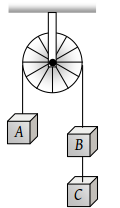(1) Zero

(2) 13 N

(3) 3.3 N

(4) 19.6 N

Concept Questions :-

Application of laws
High Yielding Test Series + Question Bank - NEET 2020

Difficulty Level:

Two masses of 4 kg and 5 kg are connected by a string passing through a frictionless pulley and are kept on a frictionless table as shown in the figure. The acceleration of 5 kg mass is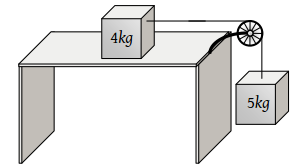(1) 49 m/s2

(2) 5.44 m/s2

(3) 19.5 m/s2

(4) 2.72 m/s2

Concept Questions :-

Application of laws
High Yielding Test Series + Question Bank - NEET 2020

Difficulty Level:

Two masses 2 kg and 3 kg are attached to the end of the string passed over a pulley fixed at the top. The tension and acceleration are

(1) $\frac{7g}{8};\text{\hspace{0.17em}}\frac{g}{8}$

(2) $\frac{21g}{8};\text{\hspace{0.17em}}\frac{g}{8}$

(3) $\frac{21g}{8};\text{\hspace{0.17em}}\frac{g}{5}$

(4) $\frac{12g}{5};\text{\hspace{0.17em}}\frac{g}{5}$

Concept Questions :-

Application of laws
High Yielding Test Series + Question Bank - NEET 2020

Difficulty Level:

Three blocks A, B and C weighing 1, 8 and 27 kg respectively are connected as shown in the figure with an inextensible string and are moving on a smooth surface. T3 is equal to 36 N. Then T2 is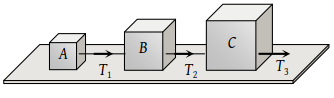(1) 18 N

(2) 9 N

(3) 3.375 N

(4) 1.25 N

Concept Questions :-

Newton laws
High Yielding Test Series + Question Bank - NEET 2020

Difficulty Level:

Two bodies of mass 3 kg and 4 kg are suspended at the ends of massless string passing over a frictionless pulley. The acceleration of the system is (g = 9.8 m/s2

(1) 4.9 m/s2

(2) 2.45 m/s2

(3) 1.4 m/s2

(4) 9.5 m/s2

Concept Questions :-

Application of laws
High Yielding Test Series + Question Bank - NEET 2020

Difficulty Level:

Three solids of masses m1, m2 and m3 are connected with weightless string in succession and are placed on a frictionless table. If the mass m3 is dragged with a force T, the tension in the string between m2 and m3 is

(1) $\frac{{m}_{2}}{{m}_{1}+{m}_{2}+{m}_{3}}T$

(2) $\frac{{m}_{3}}{{m}_{1}+{m}_{2}+{m}_{3}}T$

(3) $\frac{{m}_{1}+{m}_{2}}{{m}_{1}+{m}_{2}+{m}_{3}}T$

(4) $\frac{{m}_{2}+{m}_{3}}{{m}_{1}+{m}_{2}+{m}_{3}}T$

Concept Questions :-

Application of laws
High Yielding Test Series + Question Bank - NEET 2020

Difficulty Level:

Three blocks of masses m1, m2 and m3 are connected by massless strings as shown on a frictionless table. They are pulled with a force T3 = 40 N. If m1 = 10 kg, m2 = 6 kg and m3 4 kg, the tension T2 will be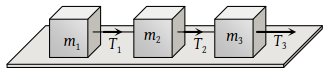(1) 20 N

(2) 40 N

(3) 10 N

(4) 32 N

Concept Questions :-

Types of forces
High Yielding Test Series + Question Bank - NEET 2020

Difficulty Level:

A light string passes over a frictionless pulley. To one of its ends a mass of 6 kg is attached. To its other end a mass of 10 kg is attached. The tension in the thread will be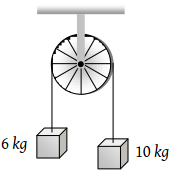(1) 24.5 N

(2) 2.45 N

(3) 79 N

(4) 73.5 N

Concept Questions :-

Application of laws
High Yielding Test Series + Question Bank - NEET 2020

Difficulty Level:

Two masses of 5kg and 10kg are connected to a pulley as shown. What will be the acceleration of the system (g = acceleration due to gravity)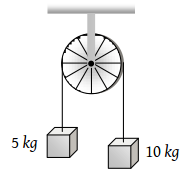(1) g

(2) $\frac{g}{2}$

(3) $\frac{g}{3}$

(4) $\frac{g}{4}$

Concept Questions :-

Application of laws
High Yielding Test Series + Question Bank - NEET 2020

Difficulty Level:

A block A of mass 7 kg is placed on a frictionless table. A thread tied to it passes over a frictionless pulley and carries a body B of mass 3 kg at the other end. The acceleration of the system is (given g = 10 ms–2)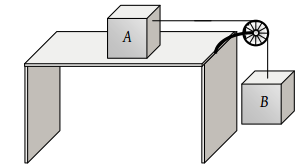(1) 100 ms–2

(2) 3 ms–2

(3) 10 ms–2

(4) 30 ms–2

Concept Questions :-

String constraint